• 符号说明： 符号 说明 ...f~=F[f]\tilde{f}=F[f]f~​=F[f] ...f1(x)f_1(x)f1​(x) 和 f2(x)f_2(x)f2​(x) 乘积的傅里叶变换等于 f1(x)f_1(x)f1​(x) 和 f2(x)f_2(x)f2​(x) 的傅里叶变换的乘积的卷积乘 12π
符号说明：

符号
说明

$\tilde{f}=F[f]$
$f$ 的傅里叶变换为 $\tilde{f}$

$f=F^{-1}[\tilde{f}]$
$\tilde{f}$ 的傅里叶逆变换为 $f$

定理：
$f_1(x)$ 和 $f_2(x)$ 乘积的傅里叶变换等于 $f_1(x)$ 和 $f_2(x)$ 的傅里叶变换的乘积的卷积乘 $\frac{1}{2\pi}$，即
$F[f_1·f_2]=\frac{1}{2\pi}F[f_1]*F[f_2]$
证明:
设 $F_{1}(\omega)=\mathcal{F}\left[f_{1}(t)\right], F_{2}(\omega)=\mathcal{F}\left[f_{2}(t)\right], \quad I \mathcal{F}$ 表示傅里叶逆变换, 则
\begin{aligned} F^{-1}\left[F_{1}(\omega) * F_{2}(\omega)\right]&=F^{-1}\left[\int_{-\infty}^{+\infty} F_{1}(\mu) F_{2}(\omega-\mu) \rm{d} \mu\right]\\ &=\frac{1}{2 \pi} \int_{-\infty}^{+\infty}\left[\int_{-\infty}^{+\infty} F_{1}(\mu) F_{2}(\omega-\mu) d \mu\right] e^{i \omega t} \rm{d} \omega\\ &=\frac{1}{2 \pi} \int_{-\infty}^{+\infty}\left[\int_{-\infty}^{+\infty} F_{1}(\mu) F_{2}(\omega-\mu) e^{i \mu t} e^{i(\omega-\mu) t} d \omega\right] \rm{d} \mu\\ &=\frac{1}{2 \pi} \int_{-\infty}^{+\infty} F_{1}(\mu) e^{i \mu t}\left[\int_{-\infty}^{+\infty} F_{2}(\omega-\mu) e^{i(\omega-\mu) t} d(\omega-\mu)\right] \rm{d} \mu\\ &=f_{2}(t) \int_{-\infty}^{+\infty} F_{1}(\mu) e^{i \mu t} \rm{d} \mu\\ &=2 \pi f_{1}(t) f_{2}(t) \end{aligned}


展开全文• 文章目录一、时域卷积定理二、频域卷积定理（时域相乘）2.1 应用：正弦幅度调制三、计算系统冲激响应 h(t) 方法3.1 频率响应 H(jω) 与系统结构关系 一、时域卷积定理 我们先给出定理：两个时域信号做卷积，就...
文章目录一、时域卷积定理二、频域卷积定理（时域相乘）2.1 应用：正弦幅度调制三、计算系统冲激响应 h(t) 的方法3.1 频率响应 H(jω) 与系统结构的关系
一、时域卷积定理
我们先给出定理：两个时域信号做卷积，就等价于它们的频谱做乘法。
即：若：$x(t)\space \underleftrightarrow{\mathscr{F}} \space X(jω); \quad y(t)\space \underleftrightarrow{\mathscr{F}} \space Y(jω)$；那么$x(t)*y(t) \space \underleftrightarrow{\mathscr{F}} \space X(jω)Y(jω)$
这给我们计算一个系统的输出带来了极大的便利。我们可以先求出输入信号的频谱和系统的频率响应，两者一乘，最后做一下傅里叶逆变换就可以得出系统的输出（时域上的）。
【Proof】：我们下面证明一下，直接从定义出发：\begin{aligned} Y(jω) &= \int_{-∞}^{+∞}y(t)e^{-jωt}dt\\ &=\int_{-∞}^{+∞}[\int_{-∞}^{+∞}x(τ)h(t - τ)dτ]e^{-jωt}dt\\\\ \end{aligned}
到这里，博主有话要说了：因为我们现在的被积函数是$x(τ)$ 和 $h(t - τ)$ 都是和 $τ$ 有关的表达式，因此我们不能简单粗暴地把它们拆开，一个是 $t$ 的积分，另一个是 $τ$ 的积分。这样是错误的！！但是我们又注意到：只有 $h(t - τ)$ 是和 $t$ 有关的，那么我们确实可以先让 $h(t - τ)$ 对 $t$ 积分，$dτ$ 放在最后面去做。
\begin{aligned} Y(jω) &=\int_{-∞}^{+∞}[\int_{-∞}^{+∞}x(τ)h(t - τ)dτ]e^{-jωt}dt\\ &=\int_{-∞}^{+∞}[x(τ)\int_{-∞}^{+∞}h(t - τ)e^{-jωt}dt]dτ \end{aligned}
这里我们需要用到傅里叶变换的延时特性：若 $x(t)\space \underleftrightarrow{\mathscr{F}} \space X(jω)$，那么 $x(t-t_0) \space \underleftrightarrow{\mathscr{F}} \space X(jω)e^{-jωt_0}$
换成数学表达式就是：$\int_{-∞}^{+∞}h(t - τ)e^{-jωt}dt = H(jω)e^{-jωτ}$
所以上式就变为：\begin{aligned} Y(jω) &=\int_{-∞}^{+∞}[x(τ)\int_{-∞}^{+∞}h(t - τ)e^{-jωt}dt]dτ\\ &=\int_{-∞}^{+∞}x(τ)e^{-jωτ}H(jω)dτ\\ &=H(jω)\int_{-∞}^{+∞}x(τ)e^{-jωτ}dτ\\ &=H(jω)X(jω) \end{aligned}
那么，问题来了：还记得我们在之前的 $Blog$ 里面说过的吗？任何一个系统都可以通过其单位冲激响应 $h(t)$ 来表征对吧，那么根据我们现在推导出的时域卷积定理：$Y(jω)=H(jω)X(jω)$，那么按理说任何一个系统应该也可以用它的频率响应 $H(jω)$ 来表征。但是，确实是这样吗？
我们知道，$H(jω)$ 是 $h(t)$ 的傅里叶变换，那么所以只有当 $h(t)$ 满足傅里叶变换的收敛条件其中之一：$\int_{-∞}^{+∞}|h(t)|^2dt < ∞\\ \space\\ \int_{-∞}^{+∞}|h(t)|dt < ∞$
那么，这个系统才会存在频率响应，也就是说，不是所有的系统都能够求出它的频率响应。那么也就不能说：任何系统都可以用它的频率响应 $H(jω)$ 来表征。但是在本文后面的部分将会给出 $h(t)$ 和 $H(jω)$（存在的情况）的计算方法。
二、频域卷积定理（时域相乘）
我们先给出定理：若 $x_1(t), x_2(t)$ 的傅里叶变换分别为：$X_1(jω), X_2(jω)$，那么有：$x_1(t)\sdot x_2(t) \space \underleftrightarrow{\mathscr{F}} \space \frac{1}{2π} X_1(jω) * X_2(jω)$
2.1 应用：正弦幅度调制
我们知道如果要计算系统的输出，我们可以通过时域卷积的方法计算得到。那么，什么场合下我们会用到时域信号相乘呢？答案就是—— 正弦幅度调制（当然不仅仅是这种，未来的 $IQ$ 调制等等也都会用得上）
我们来看看正弦幅度调制的框图：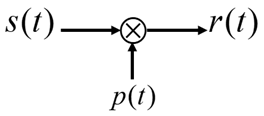其中，$s(t)$ 是我们的时域信号，$p(t) = cos(ω_0t)$，那么得到的 $r(t)$ 就是 $s(t)\sdot p(t)$
下面，我们就从频域的角度去分析正弦幅度调制：
【1】第一步：计算 $p(t)$， $s(t)$ 的频谱。
对于 $cos(ω_0t)$ 的频谱我们应该是很熟悉了，即 $P(jω) = πδ(ω+ω_0) + πδ(ω-ω_0)$，如下图所示：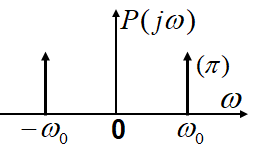我们再假设信号 $s(t)$ 的频谱如下图所示：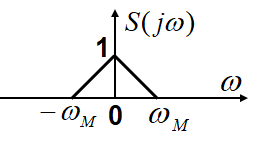根据：$x_1(t)\sdot x_2(t) \space \underleftrightarrow{\mathscr{F}} \space \frac{1}{2π} X_1(jω) * X_2(jω)$，我们可以知道两个信号相乘之后的频谱如下图所示：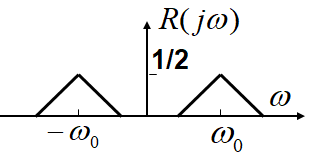这里我们得出了一个结论：信号 $s(t)$ 在时域上与 $cos(ω_0t)$ 相乘，相当于在频谱上把 $s(t)$ 的频谱一分为二之后，分别向左右各搬移 $ω_0$！ （频谱搬移）
好的，到这里，我们已经了解了正弦幅度调制的细节。可以既然有调制，那么在接收端就必然需要解调。我们如何把这个调制的信号解调出来呢？？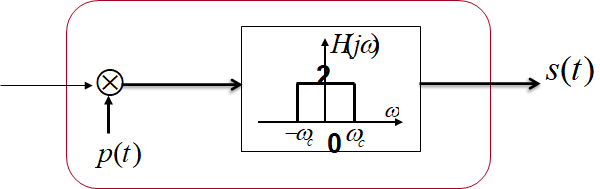我们看到，最左边的箭头的输入，就是我们刚刚那个调制的信号，在解调端，我们需要再给这个信号乘以原载波 $cos(ω_0t)$ ，然后经过一个低通滤波器（通带增益为2），就可以换原原本的信号了！
我们也是从频谱的角度来直观地看一下这个过程：
下图是输入信号 $r(t)$ 的频谱：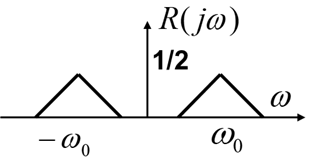下图是 $p(t)$ 的频谱，$r(t)$ 需要再和载波进行相乘：那么，二者相乘得到信号的频谱就是：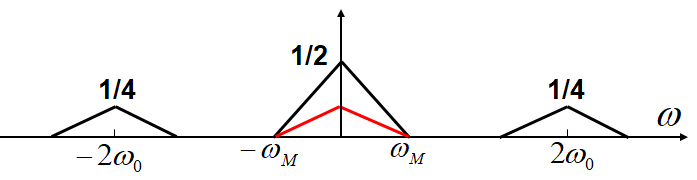此时注意：中间频谱的幅度是 $\frac{1}{2}$
那么，经过一个通带增益为2的低通滤波器，就可以恢复原信号的频谱了！（下图为该低通滤波器的频响）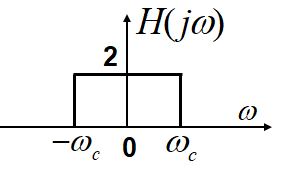三、计算系统冲激响应 h(t) 的方法
我们常常使用线性常系数微分方程来表征一个系统，如下：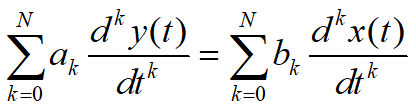这时，我们可以直接对方程两边做傅里叶变换，根据傅里叶变换的微分性可以得出：$\sum_{k=0}^{N}a_k(jω)^kY(jω) = \sum_{k=0}^Nb_k(jω)^kX(jω)$
同时，又根据：$X(jω)H(jω) = Y(jω)$，所以我们可以得到系统的频率响应为：$H(jω) = \frac{Y(jω)}{X(jω)} = \frac{\sum_{k=0}^Nb_k(jω)^k}{\sum_{k=0}^{N}a_k(jω)^k}$
【我们举一个例子来感受这种方法的简便性，同时也给大家介绍一种解题技法】：
求以下面这个微分方程表征的系统的 $h(t)$：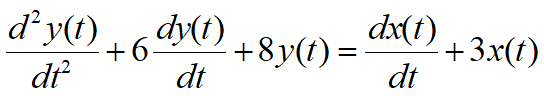两边做傅里叶变换，得：$(jω)^2Y(jω) + 6(jω)Y(jω) + 8Y(jω) = (jω)X(jω) + 3X(jω)\\ ((jω)^2 + 6(jω) + 8)Y(jω) = ((jω) + 3)X(jω)$
因此，系统得频率响应可以表示为：$H(jω) = \frac{Y(jω)}{X(jω)} = \frac{(jω)+3}{(jω)^2 + 6(jω) + 8} = \frac{jω+3}{(jω+2)(jω+4)}$
我们乍一看这个表达式看不能立刻得出结论，还需要使用下面这个技巧来分解一下：$H(jω) = \frac{A_1}{jω+2} + \frac{A_2}{jω+4} = \frac{jω+3}{(jω+2)(jω+4)}$
下面就需要计算 $A_1, A_2$。下面是固定套路：

要求 $A_1$，我们就对上面的等式两边同乘 $jω+2$，再令 $jω+2 = 0$，就可以把 $A_1$ 解出来。$A_1 = \frac{1}{2}$
要求 $A_2$ ，我们就对上面的等式两边同乘以 $jω+4$，再令 $jω+4 = 0$，就可以把 $A_2$解出来。$A_2 = \frac{1}{2}$

即：$H(jω) = \frac{1}{2}\frac{1}{jω+2} + \frac{1}{2}\frac{1}{jω+4}$
还记得单边指数信号 $e^{-at}u(t)$ 的频谱嘛？—— $\frac{1}{a+jω}$
因此，我们看到这里：$\frac{1}{jω+2}$ 的原信号就是：$e^{-2t}u(t)$。所以 $h(t)$ 为：$h(t) = \frac{1}{2}e^{-2t}u(t) + \frac{1}{2}e^{-4t}u(t)$
3.1 频率响应 H(jω) 与系统结构的关系
【1】级联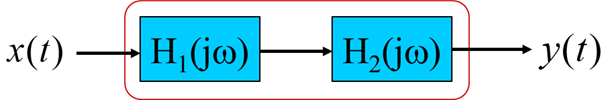还记得时域里面如果两个系统级联，那么它们的响应关系是：$h_1(t) * h_2(t)$
根据时域卷积定理，它们的频域关系就是：$H_1(jω)H_2(jω)$
【2】并联：并联里面时域和频域都一样，都是相加。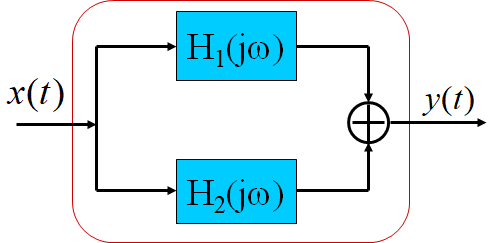$H_1(jω) + H_2(jω)$


展开全文信号处理 数字通信
• 最近在学习信号与系统这门课程，其中的一个知识点就是傅里叶变换的性质，为了更好地记忆和使用这些性质，就需要知道这些性质的证明过程，而有些性质如频域积分和频域卷积定理的证明在书中并未给出，所以在就个人理解...
傅里叶变换频域积分性质和频域卷积性质证明
最近在学习信号与系统这门课程，其中的一个知识点就是傅里叶变换的性质，为了更好地记忆和使用这些性质，最好是知道这些性质的证明过程，而有些性质如频域积分和频域卷积定理的证明在书中并未给出，所以在此对这两条性质进行证明。
1. 频域积分性质的证明
首先想要证明频域积分性质，就要先了解时域积分性质的证明，时域积分性质证明的过程如下：
$\mathcal{F}\{ \int_{ - \infty }^{ t } f(\tau)d_\tau\}=\int_{-\infty}^{\infty}\{ \int_{-\infty}^t f(\tau) d_\tau\}e^{-jwt} d_t \\ =\int_{-\infty}^{\infty}\{\int_{-\infty}^{\infty}f(\tau)\epsilon(t-\tau) d_\tau \}e^{-jwt} d_t$
经观察发现可以先将阶跃函数 $\epsilon(t-\tau)$进行傅里叶变化变成$[\pi \delta(w)+\frac{1}{jw}]e^{-jw\tau}$
$\int_{-\infty}^{\infty}f(\tau)\{\int_{-\infty}^{\infty}\epsilon(t-\tau)e^{-jwt}d_t\}d_\tau\\=\int_{-\infty}^{\infty}f(\tau)\pi \delta (w)e^{-jw\tau}d_\tau+\int_{-\infty}^{\infty}f(\tau)\cdot \frac{1}{jw}e^{-jw\tau}d_\tau\\=\pi F(0)\delta(w)+\frac{1}{jw}F(jw)$
综上时域积分性质可以表示为：若$f(t) \iff F(jw)$,则
$\int_{-\infty}^tf(\tau)d\tau \iff \pi F(0) \delta(w)+\frac{1}{jw}F(jw)$
从上述时域积分性质的证明中可以看出首先需要改变积分上限，然后变换积分次序，最后按照一般的傅里叶变换即可
这样的证明过程可以沿用到频域积分上，频域积分性质证明如下：
$\mathcal{F^{-1}}\{ \int_{ - \infty }^{ w } F(j\Omega)d_\Omega\}=\frac{1}{2\pi}\int_{-\infty}^{\infty}\{ \int_{-\infty}^w F(j\Omega) d_\Omega\}e^{jwt} d_w \\ =\frac{1}{2\pi}\int_{-\infty}^{\infty}\{\int_{-\infty}^{\infty}F(j\Omega) \epsilon(w-\Omega) d_\Omega \}e^{jwt} d_w\\=\int_{-\infty}^{\infty}F(j\Omega)\{\frac{1}{2\pi}\int_{-\infty}^{\infty}\epsilon(w-\Omega)e^{jwt}d_w\}d_\Omega\\=\frac{1}{2\pi}(\int_{-\infty}^{\infty}F(j\Omega)\pi \delta (t)e^{j\Omega t}d_\Omega+\int_{-\infty}^{\infty}F(j\Omega)\cdot \frac{j}{t}e^{j\Omega t}d_\Omega)\\=\pi f(0)\delta(t)+\frac{j}{t}f(t)$
综上频域积分性质可以表示为：若$F(jw) \iff f(t)$,则
$\int_{-\infty}^wF(j\Omega)d\Omega \iff \pi f(0) \delta(t)+\frac{j}{t}f(t)$
在以上证明过程中使用到了傅里叶变化的对称特性和移频特性
$\epsilon(t) \iff \pi \delta(w)+\frac{1}{jw}$
$对称特性：\epsilon(w) \iff \frac{1}{2\pi} (\pi \delta(-t)+\frac{1}{-jt})=\frac{1}{2\pi} (\pi \delta(t)+\frac{j}{t})$
$移频特性：\epsilon(w-\Omega) \iff \frac{1}{2\pi} (\pi \delta(t)+\frac{j}{t})e^{j\Omega t}$

2. 频域卷积性质的证明
首先还是先给出时域卷积性质的证明：
$\mathcal{F}\{f_1(t)*f_2(t)\}=\int_{-\infty}^{\infty}\{ \int_{-\infty}^{\infty} f_1(\tau)f_2(t-\tau) d_\tau\}e^{-jwt} d_t$
变换积分次序
$\mathcal{F}\{f_1(t)*f_2(t)\}=\int_{-\infty}^{\infty} f_1(\tau)\{ \int_{-\infty}^{\infty}f_2(t-\tau) e^{-jwt}d_t\} d_\tau$
因为   $f_2(t-\tau) \iff F_2(w)e^{-jw\tau}$
$\mathcal{F}\{f_1(t)*f_2(t)\}=\int_{-\infty}^{\infty}f_1(\tau)F_2(jw)e^{-jw\tau}d_\tau = F_1(jw)F_2(jw)$
所以可得性质： $f_1(t)*f_2(t) \iff F_1(jw)F_2(jw)$

模仿时域卷积定理的证明过程可得频域卷积定理的证明过程：
$\mathcal{F^{-1}}\{ F_1(jw)*F_2(jw)\}=\frac{1}{2\pi}\int_{-\infty}^{\infty}\{ \int_{-\infty}^{\infty} F_1(j\Omega)\cdot F_2(j(w-\Omega)) d_\Omega\}e^{jwt} d_w \\ =\int_{-\infty}^{\infty}F_1(j\Omega)\{\frac{1}{2\pi}\int_{-\infty}^{\infty} F_2(j(w-\Omega))e^{jwt} d_w \}d_\Omega\\=\int_{-\infty}^{\infty}F_1(j\Omega)f_2(t)e^{j\Omega t}d_\Omega\\={2\pi}(\frac{1}{2\pi}\int_{-\infty}^{\infty}F_1(j\Omega)e^{j\Omega t}f_2(t)d_\Omega)\\=2\pi f_1(t)f_2(t)$
所以可得频域卷积的性质： $\frac{1}{2\pi}f_1(t)f_2(t) \iff F_1(jw)F_2(jw)$

傅里叶变换中频域积分和频域卷积的性质已经证明完毕，如果有错误的地方，还请指正。


展开全文• 拉普拉斯锐化模板为 ... 由傅里叶卷积定理我们可以知道,空域模板中有一个频域模板与之相对应,现在空域中 左右两边同时进行傅里叶变换可得: 原理为: 结果为: (计算结果可能有错,错了麻烦指出~) ...
拉普拉斯锐化的模板为

0
-1
0
-1
5
-1
0
-1
0
由傅里叶卷积定理我们可以知道,空域模板中有一个频域模板与之相对应,现在空域中

左右两边同时进行傅里叶变换可得: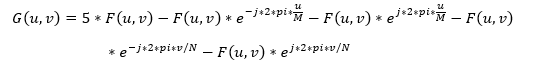原理为: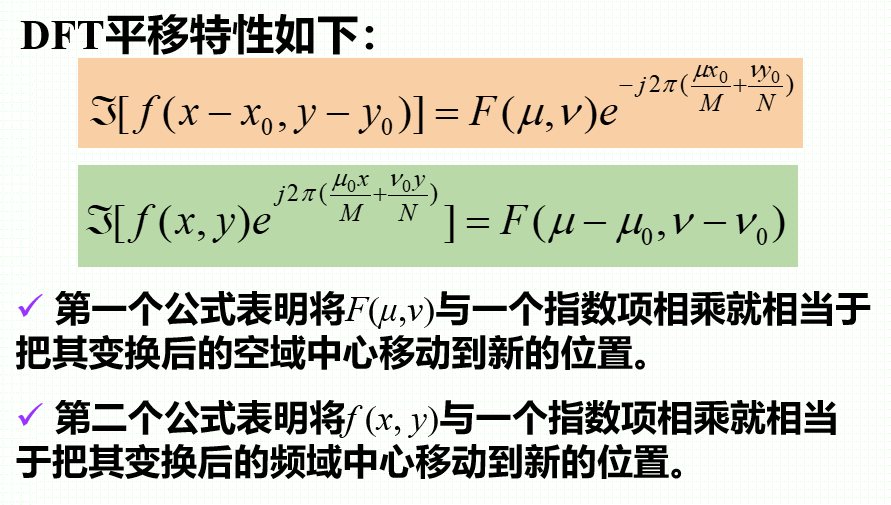结果为:

(计算结果可能有错,错了麻烦指出~)

展开全文• 文章目录傅里叶变换的性质1 线性性质2 奇偶性3 对称性4 尺度变换特性5 时移特性6 频移特性7 卷积定理8 时域微积分特性9 频域微积分特性10 相关定理 傅里叶变换的性质 意义： 傅里叶变换具有唯一性。傅里叶变换变换...
• 一日夫妻百日恩，床头吵架床尾和。要理解如何将取样后的数据还原回原函数，一定要先再...提取频域内的一个周期再把取样定理的一张图搬出来:使用一个理想低通滤波器提取一个带限函数的变换的一个周期 为原函数， 为原...
• 时域卷积定理 假设给定了两个时间函数，，的傅里叶变换为，的傅里叶变换为，则 ...频域卷积定理 假设给定了两个时间函数，，的傅里叶变换为，的傅里叶变换为，则 ，其中 傅里叶变换的基本性质 ...
• 如果一个信号为有限长度的信号，那么它导数的积分为0频域微分与积分特性卷积特性根据傅里叶变换的对偶特性，卷积定理分为时域卷积定理和频域卷积定理这两种。时域卷积表明他们对应的傅里叶变换为为乘积关系，频域...信号与系统
• ## 卷积定理的证明

千次阅读 2017-11-29 19:46:00
今天终于搞明白了卷积定理的证明，以前一直拿来就用的“时域卷积等于频域点积”终于得以揭秘： 直接证明一下连续情况好了，很容易推广到离散域（我不会）： 傅里叶变换的定义是： 　FT(f) = integrate [-inf,...
• 为了引入离散傅里叶变换，首先需要依次推导： 1，周期函数傅里叶...根据频域卷积定理可以知道： 3.2频域抽样： 函数P(w)和其时域函数p(t)： 根据时域卷积定理可以知道： 4，时间序列傅里叶...
• 时域和频域卷积定理： 提供了时域和频域转换方式，卷积是一种复杂运算，在一个域卷积转为另一个域乘法，简化运算。 什么是卷积： 时不变系统在t时刻输出，和t时刻之前历史输入s（s<t）有关，且...数据挖掘
• 文章目录傅里叶变换基础傅里叶级数傅里叶积分傅里叶变换一维连续傅里叶变换一维离散傅里叶变换二维离散傅里叶变换正变换反变换卷积卷积定理数字图像DFT空间域和频域图像频域滤波基本步骤图像频率特性分析图像滤波...
• 本文简要罗列傅里叶变换几大常用性质，方便各位复习与整理。 一、线性 若有 则必然有 二、对偶性 三、尺度变换性质 四、时移性质 五、频移性质 六、时域微分 七、卷积定理 时域卷积 频域卷积 ...信号处理
• 周期信号的频域分析 与周期模拟信号一样，周期离散信号同样可以展成傅里叶级数形式，并由此得出一新的变换对——离散傅里叶级数（Discrete Fourier Series），简记为DFS。 （一）离散傅里叶级数（DFS）的引
• 利用DFT可以大大加快卷积运算速度，因为卷积定理说明空间域卷积运算可以转换为频域的乘法运算。 ------------------------------------------------------------------------------- ...vector function
• 本文主要总结了离散傅里叶变换（DFT）定义、矩阵算法、性质，阐述了频域取样点数限制，推导了内插公式，解释了用 DFT 对连续时间信号逼近问题。在性质方面，介绍了 DFT 线性性质、如何用正变换计算逆变换、...
• 序列循环卷积运算框图 序列 的长度分别为N和M 则 证明 DFT的频域循环卷积定理 L L 7. 离散巴塞伐尔定理1 序列 的长度为N DFT 则 7. 离散巴塞伐尔定理2 证明 序列在时域计算的能量等于其在频域计算的能量 3.3 频域...
•  傅里叶变换把空间域和频域联系起来，一个空间域的序列可以通过其变换得到对应的频域的序列。而通过反变换亦能得到原始的序列。卷积定理的意义：图像增强分为频域和空间域两类。对于空间滤波来讲，对整个图像图像处理 算法
• 目录一、实验目的二、实验原理1. 连续信号的采样2.连续信号的恢复三、实验内容四、实验报告要求1、 简述实验...其傅里叶变换为，其中,设为的傅里叶变换，的频谱为，根据傅里叶变换的频域卷积定理： 若设是带限信号，带信号处理 matlab 数字通信
• 5.5 卷积、卷积定理傅里叶变换的关系及其性质_炫云云-CSDN博客 5.10 图上的傅里叶变换和逆变换_炫云云-CSDN博客 2、图卷积 在上面的基础上，利用卷积定理类比来将卷积运算，推广到Graph上。 卷积定理：函数卷积的...
• 卷积定理指出，函数卷积的傅里叶变换是函数傅里叶变换的乘积。即，一个域中的卷积相当于另一个域中的乘积，例如时域中的卷积就对应于频域中的乘积。 F(g(x)*f(x)) = F(g(x))F(f(x))其中F表示的是傅里叶变换。 这一...java
• 本文将对图像频域滤波信号与系统基本理论做一简要梳理，作为上一篇博文...包括基本信号维度与形式、复指数信号及其周期性、LSI系统、卷积定理傅里叶变换与图像频域滤波原理，以及如何构造频域滤波器等内容和源码。C++ OpenCV algorithm
• 1：傅里叶变换就是将一个信号的时域表示形式映射到一个频域表示形式， 2：换句话说，傅立叶变换的物理意义是将图像的灰度分布函数变换为图像的频率分布函数，傅立叶逆变换是将图像的频率分布函数变换为灰度分布函数...
• 卷积定理也是快速傅里叶变换算法被称为 20 世纪最重要算法之一一个原因。 快速傅里叶变换 快速傅里叶变换是一种将时域和空域中数据转换到频域上去算法。傅里叶变换用一些正弦和余弦波和来表示原函数。...
• 频域滤波演示频域滤波其实就是根据卷积定理把原来在空间域操作转移到频域，这样会提高速度，然后再傅里叶变换回去得到结果，需要注意是根据卷积定义，空域滤波时候需要翻转模板，不过一般模板都是对称...
• 卷积定理指出，函数卷积的傅里叶变换是函数傅里叶变换的乘积。即，一个域中的卷积相当于另一个域中的乘积，例如时域中的卷积就对应于频域中的乘积。 　用一个模板和一幅图像进行卷积，对于图像上的一个点，让模板的...信号处理
• Gabor变换 Gabor变换属于加窗傅立叶变换，Gabor函数可以在频域不同尺度、不同方向上...根据卷积定理，我们知道傅里叶变换可以通过卷积运算来计算得到。 卷积定理：二个二维连续函数在空间域中卷积可求其相应...
• 函数空间域的卷积的傅里叶变换是函数傅里叶变换的乘积。对应地，频率域的卷积与空间域的乘积存在对应关系。 即： 由卷积定理可知所有频域的滤波理论上都可以转化为空域的卷积操作。 给定频率域滤波器，可对其...